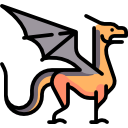# Delta: An Incremental Compiler Step 7: Bitwise Operators

## Description

Add support for integer bitwise and (&), or (|), and xor (^) binary operators. These three operators should have the same exact precedence and associativity as the addition operators. In other words, just add these operators directly to the expression production in the PEG grammar file just aside the '+' and '-' operators.

NOTE: If you’re not familiar with bitwise operators, check the corresponding wikipedia entry.

## Example

The Delta program:

31 & 42 | 5 ^ 7

should produce the following WAT code:

(module
(func
(export "_start")
(result i32)
i32.const 31
i32.const 42
i32.and
i32.const 5
i32.or
i32.const 7
i32.xor
)
)


The above WAT function’s return value should be:

8

## Unit Tests

# File: tests/test_07_bitwise.py

from unittest import TestCase
from delta import Compiler, SyntaxMistake

class TestBitwise(TestCase):

def setUp(self):
self.c = Compiler('expression_start')

def test_syntax_mistake(self):
with self.assertRaises(SyntaxMistake):
self.c.realize('1 &')

def test_and1(self):
self.assertEqual(0,
self.c.realize('10 & 5'))

def test_and2(self):
self.assertEqual(7,
self.c.realize('7 & 15'))

def test_or1(self):
self.assertEqual(15,
self.c.realize('10 | 5'))

def test_or2(self):
self.assertEqual(15,
self.c.realize('7 | 15'))

def test_xor1(self):
self.assertEqual(15,
self.c.realize('10 ^ 5'))

def test_xor2(self):
self.assertEqual(8,
self.c.realize('7 ^ 15'))

def test_mix1(self):
self.assertEqual(8,
self.c.realize('31 & 42 | 5 ^ 7'))

def test_mix2(self):
self.assertEqual(8,
self.c.realize('15 + 16 & 6 * 7 '
'| 5 * 1 ^ 1 * 7'))